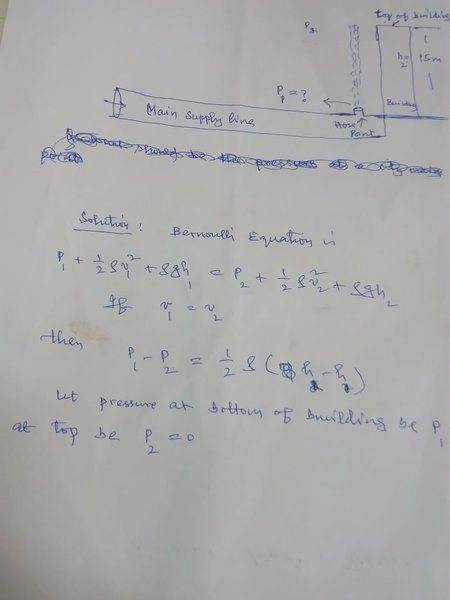# Bernoulli Equation and gauge pressure

• Zahid Iftikhar

## Homework Statement

What gauge pressure is required in the city mains for a stream from a fire hose connected to the city mains to reach a vertical building of height 15m?

## Homework Equations

Bernoulli Equation

## The Attempt at a Solution

I have tried this sum. My confusion is regarding choice of points where Bernoulli equation is to be applied. I assume two points,one at the bottom with height h1, P1 and v1, and the other at top of the building with h2=15m, P2=0 (as water is supposed to go this much height only) and v2=0 (again water is supposed to be stationary as this is the maximum height). I can't understand how is v1=v2, this is the condition that provides the required answer. Some of my work is given in the attached image please. I shall be grateful for the reply.#### Attachments

•Delta2

The velocity in the main is essentially zero, because it is a big huge pipe. What does this give you?

Maybe you should assume that the firemen are taking the hose to the top of the roof?
Thanks for the reply. Let's assume there is large sized supply pipeline that has an outlet of any cross section where pressure is required to be found as is shown by fig you added. We attach a vertical hose 15m tall to reach the top of the building,again as per fig. If the Velocities at top and bottom of the are to be same, how will pressure at bottom be minimum. In this case water may go further up. To me at top of building both pressure and velocity have to be zero. Now if we apply Bernoulli eq. then data provided is not sufficient. Pl comment.

Assuming the case #1 (you've drawn):

As Chestermiller mentioned you could assume that the main supply pipe's diameter is sufficiently large to neglect the flow velocity. Then the velocity of the initial point in the main pipe is zero and the height of the generated water fountain corresponds to the pressure difference between the main pipe and the atmospheric pressure. This of course only works, if the water jet "spreads" to fulfil the mass conservation - the jet cross section must increase to diminish the velocity.

Assuming case #2:

If the water is delivered inside a hose to the roof, then it cannot spread, therefore the flow velocity must be constant along the entire length. The flow velocity though depends on the hydraulic head (the water level in the hose), hence the height of the building.

If the pressure in the main duct corresponds exactly to the "height" of the building, in case #1 the water fountain will reach the edge of the roof. In case #2 the hose will be filled entirely with water, but none of it will exit the hose and its level will be a the nozzle. In both cases the initial velocity and the final velocity are identical zero.

•Delta2
Assuming the case #1 (you've drawn):

As Chestermiller mentioned you could assume that the main supply pipe's diameter is sufficiently large to neglect the flow velocity. Then the velocity of the initial point in the main pipe is zero and the height of the generated water fountain corresponds to the pressure difference between the main pipe and the atmospheric pressure. This of course only works, if the water jet "spreads" to fulfil the mass conservation - the jet cross section must increase to diminish the velocity.

Assuming case #2:

If the water is delivered inside a hose to the roof, then it cannot spread, therefore the flow velocity must be constant along the entire length. The flow velocity though depends on the hydraulic head (the water level in the hose), hence the height of the building.

If the pressure in the main duct corresponds exactly to the "height" of the building, in case #1 the water fountain will reach the edge of the roof. In case #2 the hose will be filled entirely with water, but none of it will exit the hose and its level will be a the nozzle. In both cases the initial velocity and the final velocity are identical zero.
Thanks a lot for the time. I need to ponder over the points you have reflected.

•Delta2